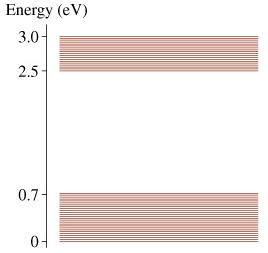# Problem: Part AWhat is the longest wavelength in the molecule’s absorption spectrum?= ? nmPart BWhat is the shortest wavelength in the molecule’s absorption spectrum?= ? nmPart C ( I tried 2486 nm and 1776 nm but was incorrect)What is the longest wavelength in the molecule’s fluorescence spectrum?= ? nmPart D ( I tried 413 nm, but it was incorrect 12400/3.0= 4133.33)What is the shortest wavelength in the molecule’s fluorescence spectrum?= ? nm

###### FREE Expert Solution

The change in the energy of the molecule is given by:

$\overline{){\mathbf{∆}}{\mathbf{E}}{\mathbf{=}}\frac{\mathbf{h}\mathbf{c}}{\mathbf{\lambda }}}$

92% (385 ratings)###### Problem DetailsPart A
What is the longest wavelength in the molecule’s absorption spectrum?
= ? nm

Part B
What is the shortest wavelength in the molecule’s absorption spectrum?
= ? nm

Part C ( I tried 2486 nm and 1776 nm but was incorrect)
What is the longest wavelength in the molecule’s fluorescence spectrum?
= ? nm

Part D ( I tried 413 nm, but it was incorrect 12400/3.0= 4133.33)
What is the shortest wavelength in the molecule’s fluorescence spectrum?
= ? nm